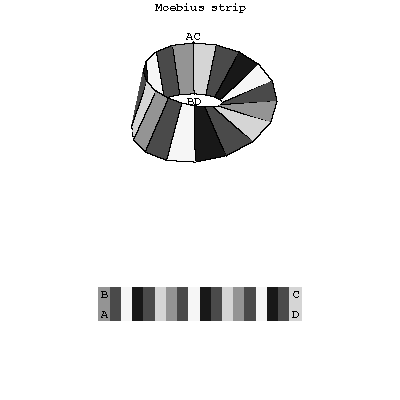# Möbius strip

A non-orientable surface with Euler characteristic zero whose boundary is a closed curve. The Möbius strip can be obtained by identifying two opposite sides $AB$ and $CD$ of a rectangle $ABCD$ so that the points $A$ and $B$ are matched with the points $C$ and $D$, respectively (see Fig.).Figure: m064310a

In the Euclidean space $E^3$ the Möbius strip is a one-sided surface (see One-sided and two-sided surfaces).

The Möbius strip was considered (in 1858–1865) independently by A. Möbius and I. Listing.

How to Cite This Entry:
Möbius strip. Encyclopedia of Mathematics. URL: http://www.encyclopediaofmath.org/index.php?title=M%C3%B6bius_strip&oldid=31555
This article was adapted from an original article by A.B. Ivanov (originator), which appeared in Encyclopedia of Mathematics - ISBN 1402006098. See original article